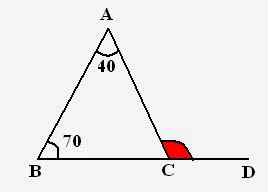# Geometry -concepts and properties for SSC CGL Tier-I Geometry is one of the most important topics of Quantitative Aptitude section of SSC CGL exam. ItYork 2020-11-30 Answered
Geometry -concepts and properties for SSC CGL Tier-I Geometry is one of the most important topics of Quantitative Aptitude section of SSC CGL exam. It includes various concepts related to lines, angles, triangles, circles, polygons and so on. So, today I will just discuss concept and properties of triangle. Always remember that in Geometry, You need a very basic understanding. Cramming is not gonna help you anywhere.
You can still ask an expert for help

• Questions are typically answered in as fast as 30 minutes

Solve your problem for the price of one coffee

• Math expert for every subject
• Pay only if we can solve itquestion2answer

## Triangles

$\mathrm{△}=\text{Figure with three sides}$.  Study the following triangle: A,B,C to points. a, b, c to sides. x, y, z to angles.Perimeter of triangle $=a+b+c$
Remember that, Sum of all the angles is always ${180}^{\circ }$
i.e. $x+y+z={180}^{\circ }$

### Classification of Triangles

Basically there are three types of triangles excluding right angle triangle. Let me tell you how they vary from each other.

• Scalene Triangle
• Isosceles Triangle
• Equilateral Triangle

Scalene Triangle: No side of triangle is equal.
Isosceles Triangle: Two sides of triangle are equal.
Equilateral Triangle: All sides of triangle are equal.

 Scalene Isosceles Equilateral Definition a ne b ne c ane b = c a = b = c Area A $\left(b/4\right)\sqrt{4{a}^{2}–{b}^{2}}$ $\left(3{\right)}^{\frac{1}{2}}\left({a}^{2}\right)/4$ Height - $\sqrt{\left(4{a}^{2}-{b}^{2}\right)/2}$ $\left(3{\right)}^{\frac{1}{2}}a/2$

$A={s\left(s-a\right)\left(s-b\right)\left(s-c\right)}^{1/2}$
where, $s=\left(a+b+c\right)/2$

Properties of external angles of Triangle:

1. Sum of all exterior angles is ${360}^{\circ }$
Study the following set of triangles and their exterior angles,a, b, c to Interior angles. p, q, r and s, t, u to Exterior angles.
So, sum of exterior angles $={360}^{\circ }$ i.e. $p+q+r={360}^{\circ }$ and $s+t+u={360}^{\circ }$
2. Next property of exterior angle which is important in paper point of view:

External angle = Sum of two internal angles.

For example: In above figures,
$r=a+b$
$q=a+c$
$s=b+c$ and so on.

## Right angle Triangle

Following triangle is a right angle triangle i.e. a triangle with one out of three ${90}^{\circ }$ angle.### Area of right angle triangle

Area$=\frac{1}{2}×$ Base times Perpendicular

### Example with Solution

Example: In following figure, CE is perpendicular to AB, angle $ACE={20}^{\circ }$ and angle $ABD={50}^{\circ }$. Find angle BDA:Solution: To Find: angle BDA
For this what we need --- angle BAD  Because, Sum of all angles $={180}^{\circ }$
Consider, triangle ECA,
$CEA+EAC+ACE={180}^{\circ }$ i.e. ${90}^{\circ }+{20}^{\circ }+EAC={180}^{\circ }$ Therefore, $EAC={70}^{\circ }$
Now, come to triangle ABD,
$ABD+BDA+BAD={180}^{\circ }$

${70}^{\circ }+{50}^{\circ }+BAD={180}^{\circ }$

Therefore, $BAD={60}^{\circ }$
Example: In given figure. BC is produced to D and angle $BAC={40}^{\circ }$ and angle $ABC={70}^{\circ }$. Find angle ACD:Solution: In above figure, ACD is an exterior angle, and according to property, Exterior angle = Sum of interior angles Therefore, $ACD={70}^{\circ }+{40}^{\circ }={110}^{\circ }$
This is not the end of this chapter. These are just the basics. In next session, I will discuss some important results, properties (congruency, similarity) and much more. Always remember, Geometry needs practice and time.